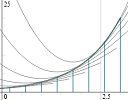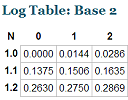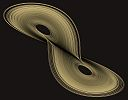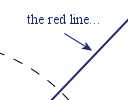Search IntMath
Close

# IntMath Newsletter: Latest applets, visual math

By Murray Bourne, 31 Jul 2014

31 Jul 2014

1. Latest interactive math applets
2. Resource - Context Free math-based art
3. Color Blindness graph simulator
4. IntMath Poll results
5. Math puzzles
6. Final thought - What is the point of education?

## 1. Latest interactive math applets

### a. New Riemann Sums appletI recently added a new Riemann Sums applet to IntMath. It allows you to explore the concept of finding areas under curves. Go to: Riemann Sums Applet You can see how we find areas under curves via rectangles, using an interactive applet.

Here's some background on this important calculus topic:

Riemann Sums background

### b. Interactive log table and calculator

This will bring back memories for older readers.A subscriber asked if I knew of a log table with 9 decimal place accuracy. (Traditionally, log books came with 4 decimal place accuracy.) I didn't, and so I created an interactive log calculator, which works in several different bases (2, e, 10, 20 and 100). You can input any (positive) value and it gives you the log value. See it at: Interactive Logarithm Table.

There's also an interactive log table which gives output up to 9 decimal places.

You may be wondering, why create electronic log tables when the real thing disappeared 30 years ago? Well, we can still learn from exploring such tables, in a way that an ordinary calculator cannot. And I don't know too many hand-held calculators that can work in base 2, 20 and 100!

## 2. Context Free math-based art

Here's an idea for something interesting to do during the summer break. Create some math-based art, and learn some STEM skills in the process!Context Free is software you can use to produce some beautiful math-based art. Build STEM skills while triggering your creative side. Context Free math-based art

## 3. Color Blindness graph simulator

I wrote an article a few years ago about Color blindness and math.

My main point in that article was that math teachers should make sure their diagrams, charts and graphs can be understood by the 8% or so of male students who do not perceive the full color range.The following link is to a JSXGraph graph that has different color lines, points and regions. You can use the buttons at the the top to test what the graph would look like for people with various types of color blindness.

## 4. Poll results

### What type of math is going to be most useful for you in the future?

It looks like calculus is the most useful type of math for many of IntMath's readers, just ahead of algebra. It's unfortunate trigonometry is seen as least useful, since there are many Uses of Trigonometry.

Poll results:

 Calculus 27% Algebra 22% Financial math 21% Statistics 12% Geometry 11% Trigonometry 7%

Current poll: The latest poll asks which financial math topic will have the most impact on your life.

You can respond to this poll question on any (inner) page of IntMath, for example this one: World Population Live.

## 5. Math puzzles

The puzzle in the last IntMath Newsletter asked about the probability of the numbers on balls in a box being relatively prime. There was only one correct answer with explanation, by Tomas. Tomas made some assumptions before tackling the problem. This is something we often need to do in "real world" math problems. Nicos' answer was close!

New math puzzle: The Phollee pyramid of ancient Egypt had a square base, but the four triangular faces were all of different shapes and size. The lengths of three of the sloping edges were 150, 160 and 200 m. The 150 m and 160 m edges were not adjacent. How long was the fourth edge?

## 6. Final thought: The point of education?

For some of you, education is vital for your future. It is valued by your society and considered worthy of full effort. You feel as a result of your learning that you are growing as a person, your horizons are wider and you understand more about the world.

Sadly, for others, it is a "necessary evil", something that they need to survive when young, and get away from as soon as possible.

Arthur W. Forshay, director of the Bureau of Educational Research at Ohio State University, wrote:

"The one continuing purpose of education, since ancient times, has been to bring people to as full a realization as possible of what it is to be a human being."

What does your education mean to you?

Until next time, enjoy whatever you learn.

### Comment Preview

HTML: You can use simple tags like <b>, <a href="...">, etc.

To enter math, you can can either:

1. Use simple calculator-like input in the following format (surround your math in backticks, or qq on tablet or phone):
a^2 = sqrt(b^2 + c^2)
(See more on ASCIIMath syntax); or
2. Use simple LaTeX in the following format. Surround your math with $$ and $$.
$$\int g dx = \sqrt{\frac{a}{b}}$$
(This is standard simple LaTeX.)

NOTE: You can mix both types of math entry in your comment.